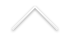Numerical Analysis is the study of the algorithms used for solving the problems of Continuous Mathematics. One of its main branches is the study of numerical solutions to Partial Differential Equations. Several PDEs of interest are hard or even impossible to be solved analytically, but numerical methods are able to find approximate solutions where the error can be estimated and controlled.

The Institute of Mathematics at UFRJ has an active researching group on this field, with several related research lines:

• Weighted essentially non-oscillatory schemes (WENO) for hyperbolic conservation laws;
• Numerical approximation of PDEs using finite element methods (FEM);
• Oil reservoir simulations.

Researchers:

• Bruno Costa
• Flavio Dickstein
• Henrique Versieux
• Monique Carmona
• Paulo Goldfeld